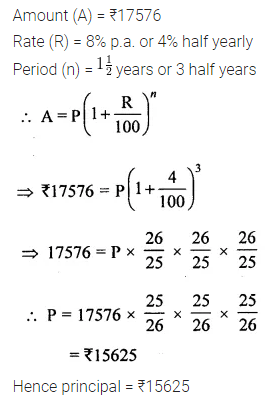# ML Aggarwal Class 8 Solutions for ICSE Maths Model Question Paper 2

## ML Aggarwal Class 8 Solutions for ICSE Maths Model Question Paper 2

Choose the correct answer from the given four options (1-2):
Question 1.
If 295×703 is divisible by 11, then value of x is
(a) 5
(b) 6
(c) 7
(d) 8
Solution:Question 2.
If C.P. of an article is ₹500 and S.P. is ₹600, then, his profit % is
(a) 10%
(b) 15%
(c) 20%
(d) 25%
Solution:Question 3.
Find the values of the letters in the following and give reasons for the steps involved: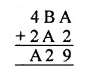Solution:Question 4.
Ramu bought a fan for ₹1080 including 8% VAT. Find the price of fan before VAT was added.
Solution: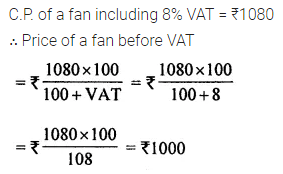Question 5.
Find the amount and compound interest on ₹2000 for 2 years at 5% per annum, interest payable yearly.
Solution:Question 6.
If P = {letters of the word HYDERABAD} and Q= {letters ofthewordALLAHABAD}, find
(i) P ∪ Q
(ii) P ∩ Q
Also verify that
n(P ∪ Q) = n(P) + n(Q) – n(P ∩ Q).
Solution:Question 7.
A blanket was marked for ₹500. A shopkeeper allows a discount of 10%. But due to partial damage in the blanket he has to give an extra discount of 20%. Find the S.P. of the blanket.
Solution: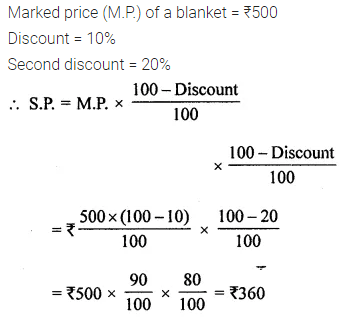Question 8.
In a 3 digit number unit’s digit, ten’s digit and hundred’s digit are in the ratio 3 : 5 : 7. If the difference of original number and number obtained by reversing the digits is 396, find the number.
Solution: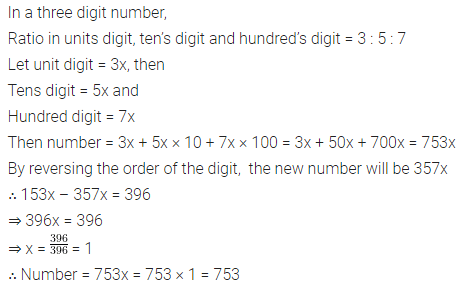Question 9.
In an exam atleast 40% marks are required to pass the exam. Rohit uses unfair means to pass the exam but fails by 20 marks. If he scored 220 marks, then find the maximum marks.
Is using unfair means in exam is good? Why should we not use unfair means?
Solution: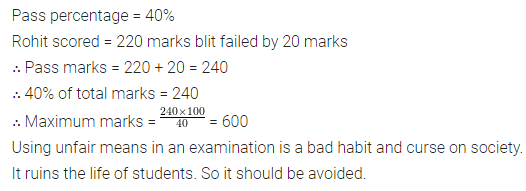Question 10.
Find the sum invested for $$1 \frac{1}{2}$$ years compounded half-yearly at the rate of 8% p.a. that will amount to ₹ 17576.
Solution: